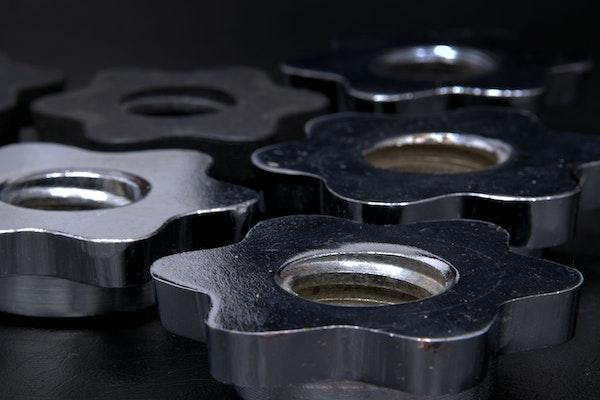# 8 Top Factors Affecting the Speed of an Electric Linear ActuatorThe speed of an electric linear actuator depends on many factors, including its friction, load profile, shaft radius, pulley radius, gearing arrangement, applied voltage, and current density. These factors affect different aspects of this machine, such as the torque-speed ratio curve or acceleration and deceleration time constants. Here is a list of factors affecting the speed of an electric linear actuator.

## Applied Voltage

Voltage is the ability of a conductor to carry an electric current away from or back to another circuit element. For example, in an electric linear actuator, voltage is applied across the power lead, creating the load’s motion through electric motors. The voltage that is applied across the power lead can be in either direct current or alternating current.

## Current Density

Current density is the rate of charge flow through a conductor. It is expressed in terms of amperes per square meter. It is the amount of electric current per unit area or quantity corresponding to the electric charge passing through a cross-sectional area per second.

The current density is held in the machine running at a given speed and is usually constant for a given voltage and frequency. The electric linear actuator can be designed to run at different speeds.

## Gearing Arrangement

The gearing arrangement of the electric linear actuator strongly affects the speed of this machine. There are two main types of gearing arrangements in linear actuators. These are parallel and series gearing arrangements. Parallel gearing is the most common design for these machines, where two or more gears or pulleys can be used in a straight line to transmit torque from an input shaft to an output.

## Friction

The friction affects the overall performance of a linear actuator as it affects its acceleration and deceleration time constants and its mechanical losses. The friction that is present in this machine is mainly generated due to wear, bearing resistance, and power transmission losses.

The load profile describes the shape of the load that causes the electric linear actuator to move and convert electrical energy into motion. This machine mainly has two types of loads, obvious and embedded loads. The embedded load exists inside the wireline while enclosed in casing, tubing, or hole.

The pulley radius is responsible for increasing or decreasing the speed of an electric linear actuator. The pulley radius is directly proportional to the angular, tangential, and ratio between input and output torque.

## The Drive Mechanism

The drive mechanism is the arrangement of gears and pulleys used to transfer torque from an electric motor into a load, affecting the speed of the linear actuator. There are three types of drive mechanisms used in electric linear actuators. They are the chain drive mechanism, belt drive mechanism, and worm gear drive mechanism.

These mechanisms can be used to create a variety of gearing arrangements. However, the drive mechanism must have friction and mechanical losses to maintain the internal drum from slipping on the load, regardless of load changes.

## The Motor

The motor is a device that converts electrical energy into mechanical energy through the rotation of a magnetic field. It consists of magnets divided into loops, poles, or segments. The electric linear actuator transfers power from an electric motor to the internal drum, which is responsible for increasing or decreasing the speed of an electric linear actuator.

## The Bottom Line

An electric linear actuator is a machine that can convert electrical energy into motion. It can transmit the motion to any load through a connecting mechanism or power chain. Electric motors power the electric linear actuator, and it is a kind of transmission unit between the motor and the load.

The speed of the electric actuators linear mainly depends on the above factors. These factors that cause the machine to be fast or slow are mostly interrelated and interchangeable. One factor can easily be affected by another factor.

Scroll to Top